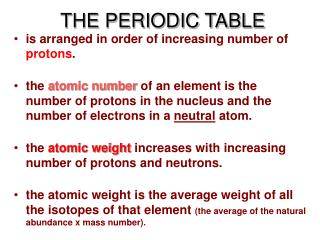DownloadDownload PresentationTHE PERIODIC TABLE

# THE PERIODIC TABLE

Télécharger la présentation## THE PERIODIC TABLE

- - - - - - - - - - - - - - - - - - - - - - - - - - - E N D - - - - - - - - - - - - - - - - - - - - - - - - - - -
##### Presentation Transcript

1. THE PERIODIC TABLE • is arranged in order of increasing number of protons. • the atomic number of an element is the number of protons in the nucleus and the number of electrons in a neutral atom. • the atomic weight increases with increasing number of protons and neutrons. • the atomic weight is the average weight of all the isotopes of that element (the average of the natural abundance x mass number).

2. THE PERIODIC TABLE • is divided into metals(left side), nonmetals(right side), and metalliods. • is arranged in rows (across) in order of increasing energy levels of valence electrons (called periods). The period number represents the energy level for that row. • is arranged in columns (down) in order of similar orbitals for the valence electrons (called groups). • The elements are represented by one or two letter symbols, which have some correlation to the element name.

3. Metals Located on the left side of the periodic table Tends to lose electrons to form cations Has low ionization energies & electronegativity values Forms compounds with nonmetals but not other metals Good conduction of heat and electricity Lustrous & malleable solids excepts Hg which is a liquid Ductile Nonmetals Located on the right side of the periodic table Tends to gain electrons to form anions Has high ionization energies & electronegativity values Forms compounds with both metals and nonmetals Poor conduction of heat and electricity Non-lustrous & brittle or gaseous Non-ductile THE PERIODIC TABLE

4. THE PERIODIC TABLE • is divided into "blocks" which represent the orbital the electron occupies; s, p, d,andf. • is read left to right; 1s, 2s, 2p, 3s, 3p, 4s.... • is arranged as representative elements (s and p block elements), transition metals (d block metals), lanthanidesandactinides (f block metals). • is arranged in order of increasing energy of the subshells; the lowest energy level is always filled first. • the noble gases are unreactive since they have a completely filled shell and are the lowest in energy.

5. THE PERIODIC TABLE • Groups or families display similar physical and chemical reactivity. • the group number (roman numerals) on top represents the number of valence electrons available for that column. • common groups are: group I = alkali metals group II = alkaline earth metals group VI = chalogens group VII = halogens group VIII = noble or inert gases

6. THE PERIODIC TABLE • demonstrates the trends in atomic properties; the periodicityof the elements. • Atomic radius, • ionization energy, • electronegativity, • magnetism are some of the properties of atoms which can be predicted using the periodic table.

7. THE PERIODIC TABLE decreases • Atomic radius In general, the atomic radii of the elements within a row (period)decreases from left to right due to an increase in nuclear charge whereas the radii increases down a column(group) due to the addition of electrons in higher energy levels. increases

8. THE PERIODIC TABLE increases • Electronegativity Electronegativity describes the ability of an atom to attract electrons to itself in a chemical bond. It is the attractive force that one atom has for a shared pair of electrons of a different atom in a covalent bond. Notice that the electronegativity can be related to the ionization energy and electron affinity. The electro-negativity generally increases across a period and decreases down a group. decrease

9. PRACTICE PROBLEMS about the PERIODIC TABLE Classify the elements as representative, transition, lanthanide, or actinide. 1. Cd 2. Te 3. Sr 4. Al 5.Gd 6. Rh 7. Cf 8. Be 9. Pd 10. O Classify the elements by the group name: 1. Be 2. Rb 3. Xe 4. Se 5. Br Arrange in order of increasing electronegativity. C O Be N Sr S B Arrange in order of increasing atomic radius. C O Be N Sr S B

10. ANSWERS Classify the elements as representative, transition, lanthanide, or actinide. 1. Transition 2. Representative 3. representative 4. representative 5. lanthanide 6. transition 7. actinide 8. representative 9. transition 10. Representative Classify the elements by the group name: 1. Alkaline earth 2. alkali 3. noble 4. chalogen 5. halogen Arrange in order of increasing electronegativity. Sr < Be < B < S = C < N < O Arrange in order of increasing atomic radius. O < N < C < S < B < Be < Sr